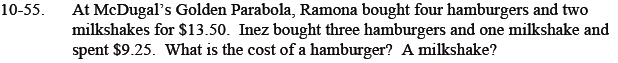Home > CCA2 > Chapter 10 > Lesson 10.1.3 > Problem10-55

10-55.

At McDugal’s Golden Parabola, Ramona bought four hamburgers and two milkshakes for $13.50. Inez bought three hamburgers and one milkshake and spent$9.25. What is the cost of a hamburger? A milkshake? Homework Help ✎Write an equation for each sentence.
You should have two equation with two variables.
Solve the system of equations.

4H + 2M = \$13.50

Now write the other equation and solve the system.